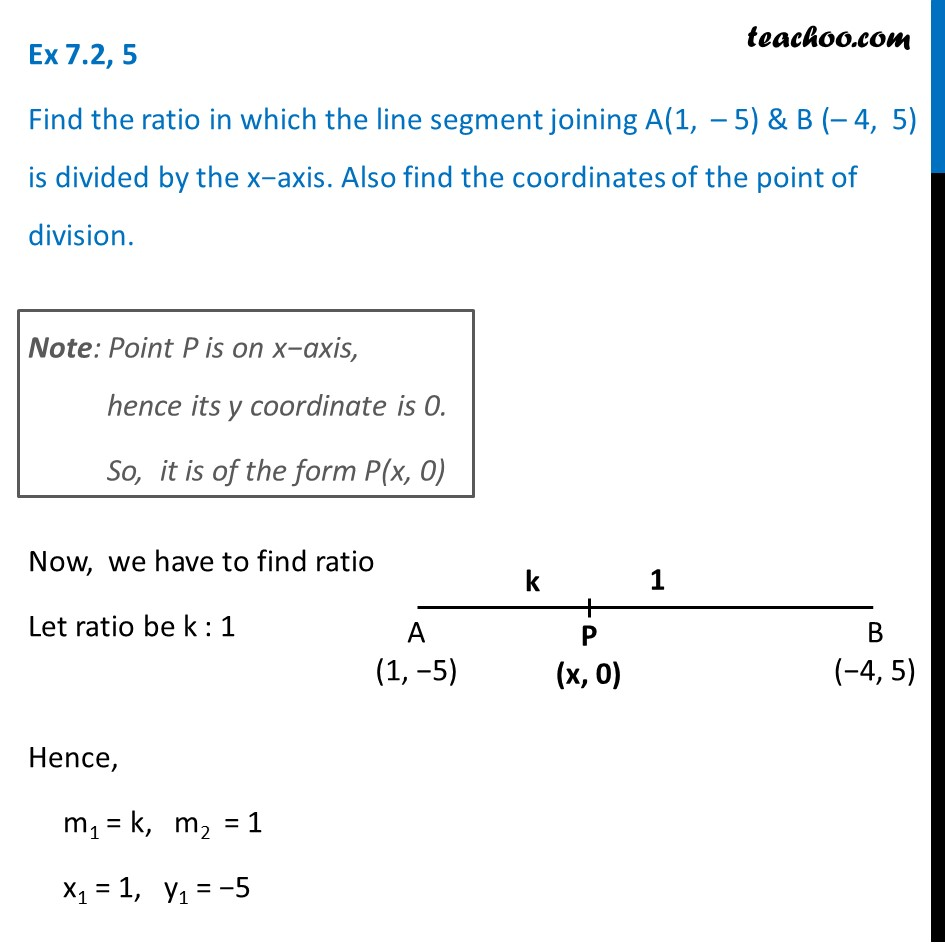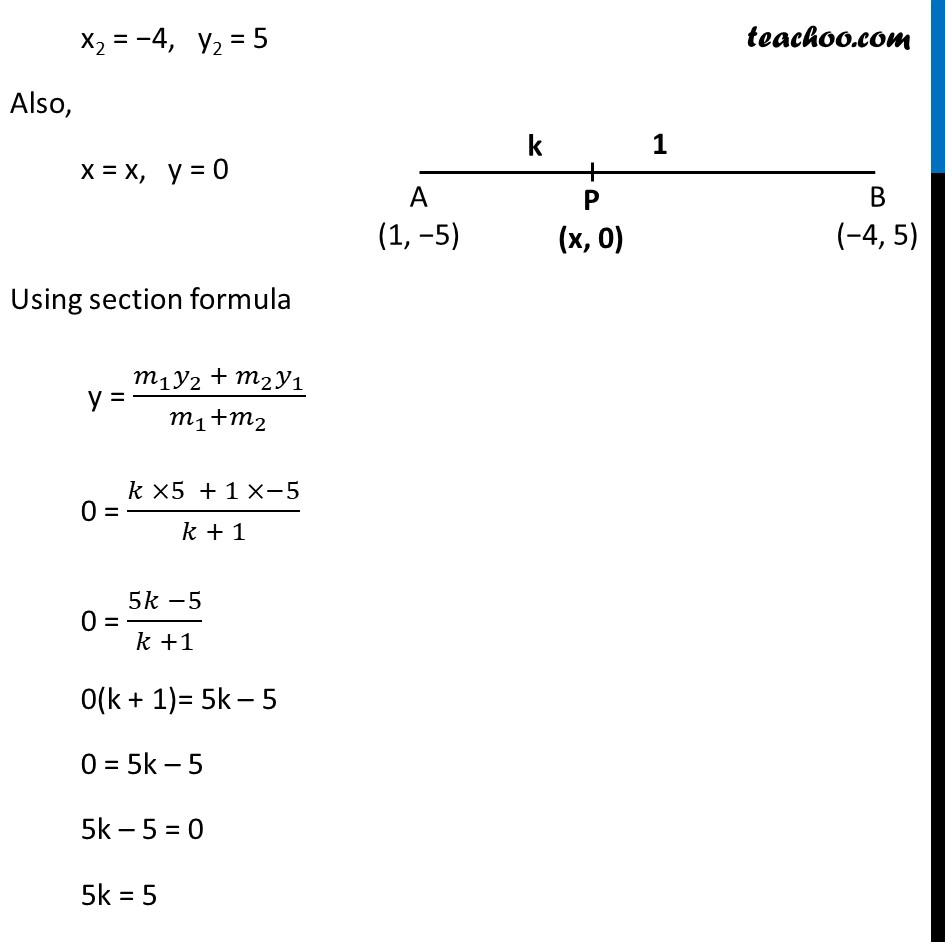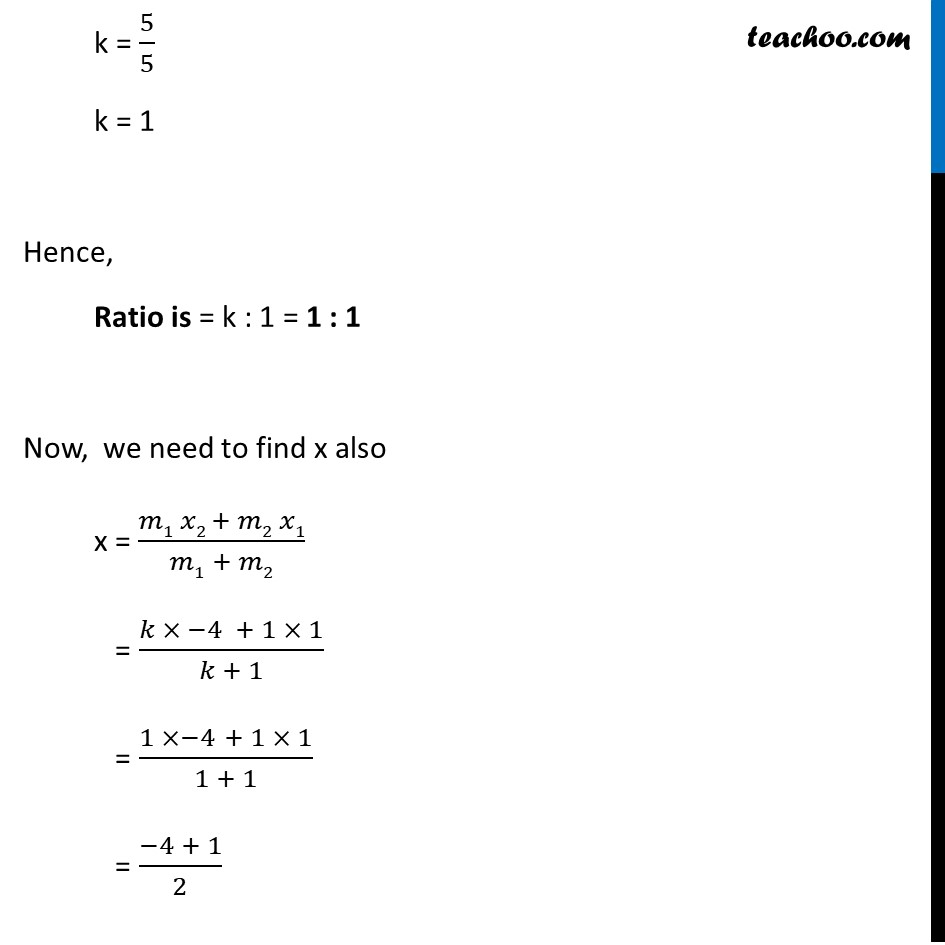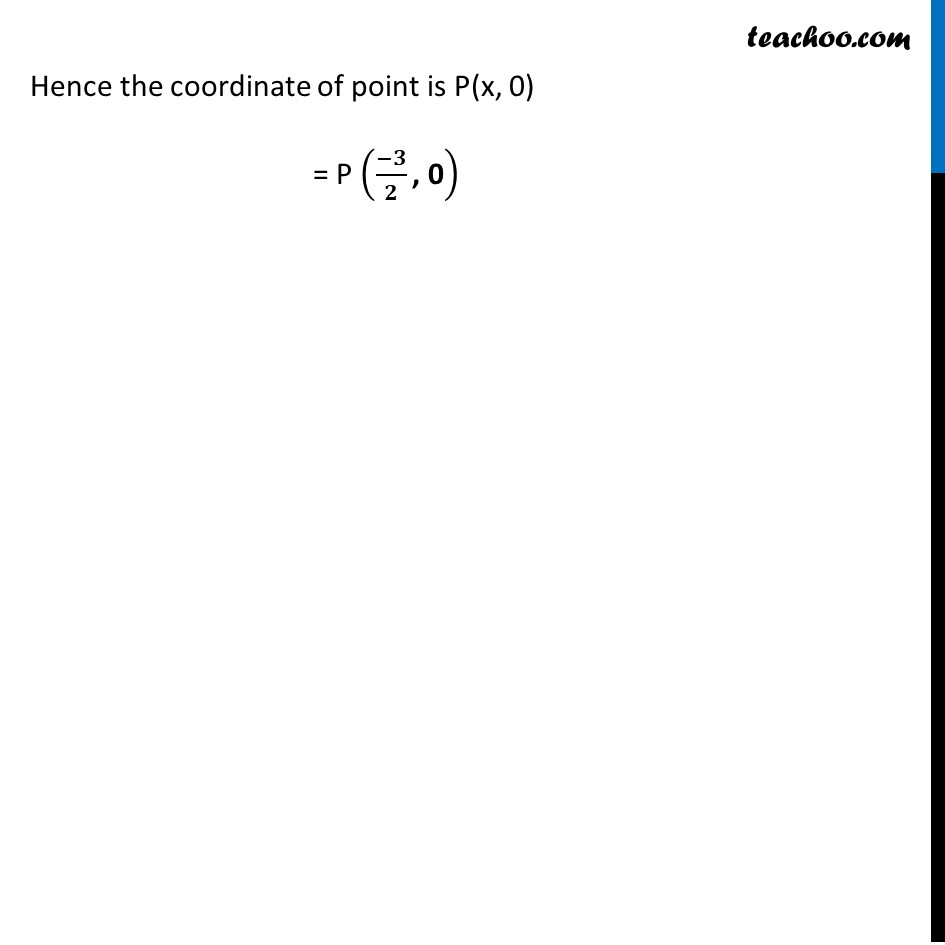Finding ratio

Chapter 7 Class 10 Coordinate Geometry
Concept wiseSolve all your doubts with Teachoo Black (new monthly pack available now!)

### Transcript

Ex 7.2, 5 Find the ratio in which the line segment joining A(1, – 5) & B (– 4, 5) is divided by the x−axis. Also find the coordinates of the point of division. Now, we have to find ratio Let ratio be k : 1 Hence, m1 = k, m2 = 1 x1 = 1, y1 = −5 Note: Point P is on x−axis, hence its y coordinate is 0. So, it is of the form P(x, 0) x2 = −4, y2 = 5 Also, x = x, y = 0 Using section formula y = (𝑚_1 𝑦_2 + 𝑚_2 𝑦_1)/(𝑚_1+𝑚_2 ) 0 = (𝑘 ×5 + 1 ×−5)/(𝑘 + 1) 0 = (5𝑘 −5)/(𝑘 +1) 0(k + 1)= 5k – 5 0 = 5k – 5 5k – 5 = 0 5k = 5 k = 5/5 k = 1 Hence, Ratio is = k : 1 = 1 : 1 Now, we need to find x also x = (𝑚1 𝑥2 + 𝑚2 𝑥1)/(𝑚1 + 𝑚2) = (𝑘 × −4 + 1 × 1)/(𝑘 + 1) = (1 ×−4 + 1 × 1)/(1 + 1) = (−4 + 1)/2 = (−3)/2 Hence the coordinate of point is P(x, 0) = P ((−𝟑)/𝟐 ", 0" )